﻿ 第35课 函数对象分析

### 第35课 函数对象分析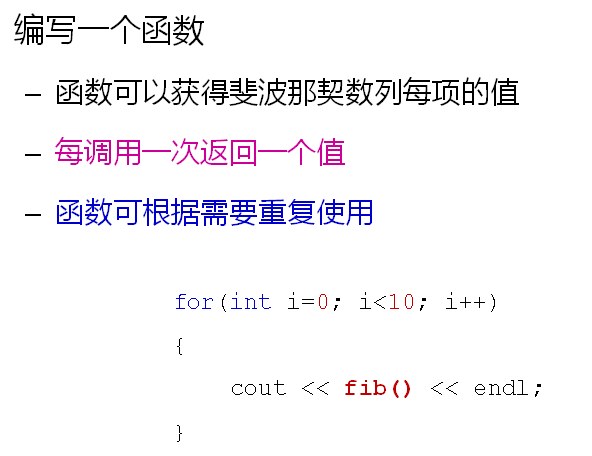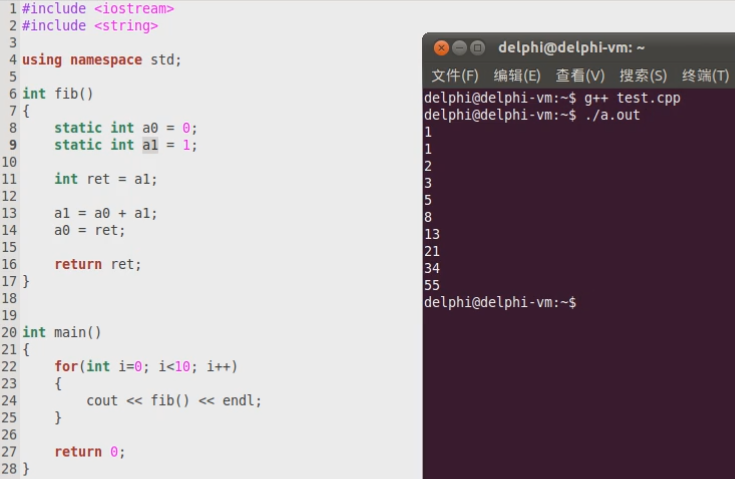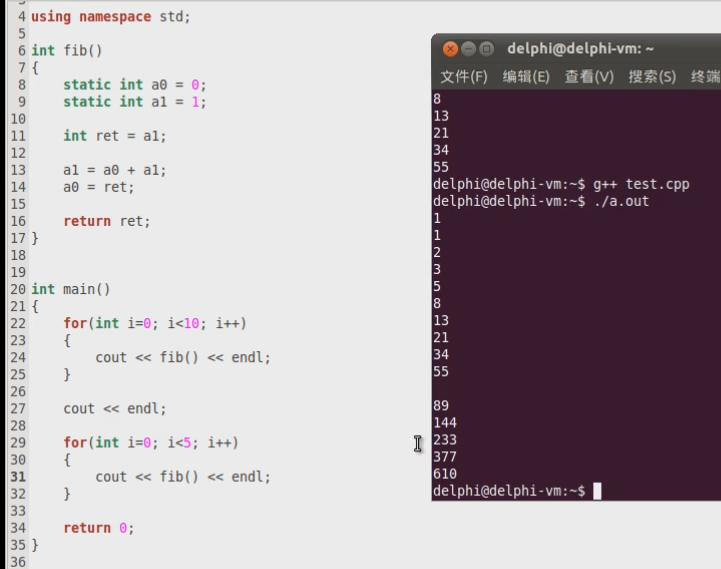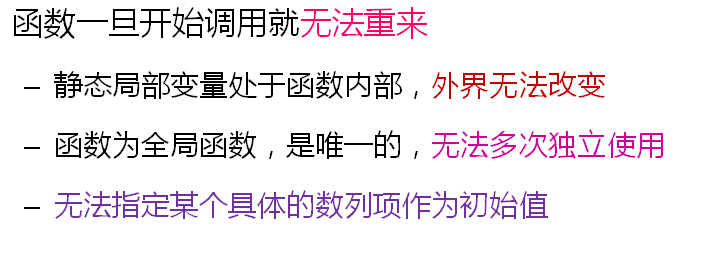怎么具体完成这项工作呢，那就是操作符重载了。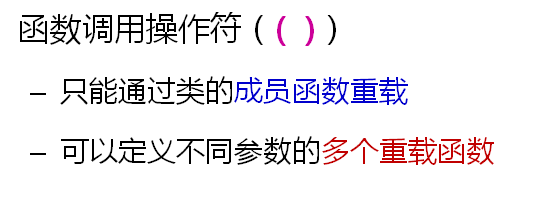()操作符是编译器内置的操作符，它的地位和数组访问操作符[]是一致的。

``` 1 #include <iostream>
2 #include <string>
3
4 using namespace std;
5
6 class Fib
7 {
8     int a0;
9     int a1;
10 public:
11     Fib()
12     {
13         a0 = 0;
14         a1 = 1;
15     }
16
17     Fib(int n)
18     {
19         a0 = 0;
20         a1 = 1;
21
22         for(int i=2; i<=n; i++)
23         {
24             int t = a1;
25
26             a1 = a0 + a1;
27             a0 = t;
28         }
29     }
30
31     int operator () ()
32     {
33         int ret = a1;
34
35         a1 = a0 + a1;
36         a0 = ret;
37
38         return ret;
39     }
40 };
41
42 int main()
43 {
44     Fib fib;
45
46     for(int i=0; i<10; i++)
47     {
48         cout << fib() << endl;
49     }
50
51     cout << endl;
52
53     for(int i=0; i<5; i++)
54     {
55         cout << fib() << endl;
56     }
57
58     cout << endl;
59
60     Fib fib2(10);
61
62     for(int i=0; i<5; i++)
63     {
64         cout << fib2() << endl;
65     }
66
67     return 0;
68 }```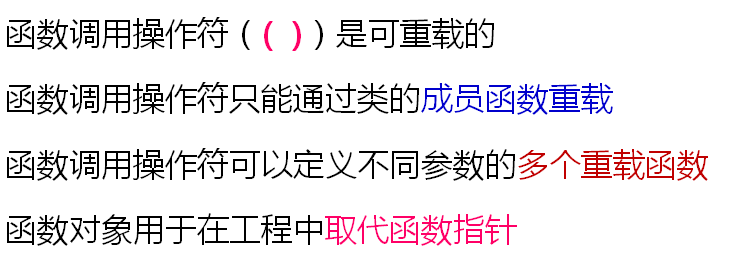在C++的工程中尽量少用原生指针，我们使用string对象取代字符串，对于数组我们可以使用数组对象取代，对于函数指针就可以用函数对象取代。